# 欧拉及拉格朗日下的动量方程压力项竟然不一样

• 算例一直发散，整不明白。今天看了半天代码，跟方程对不上，发现差了一个相分数。很奇怪。于是翻了几个文献，发现

Eulerian-Eulerian和Eulerian-Lagrangian下的动量方程压力项竟然不一样

# Eulerian-Lagrangian压力项不需要乘以相分数

主要看压力项，取自三篇不同的SCI，没有相分数！才发现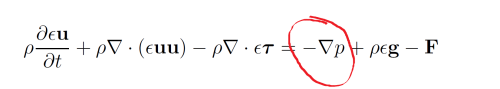# Eulerian-Eulerian压力项需要乘以相分数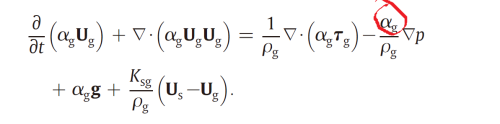.•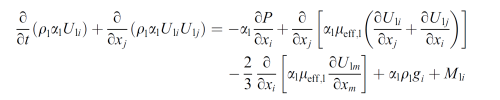上面应该是某文章里面有个笔误的Eulerian-Lagraigian方程，应该没有相分数。
有误，见下文。

植入代码的时候要小心，如果方程有笔误的话，怎么可能收敛呢？不过我自己的文章也有很多笔误，没办法，方程太多..• 昨天在植入算法的时候（参考多相流的数学模型里面的方程），发现最后推导出来的压力泊松方程里面带一个$\alpha_\rc^2$ 。之前从来没见过带平方的压力泊松方程于是回头仔细仔细再仔细的看方程，看是不是哪里错了。

到头来，发现最开始的连续相动量方程文献里面竟然不一样，就是上文说的，压力梯度项相差一个相分数$\alpha_\mathrm{c}^2$。此处不赘述。昨晚上搞完之后就出去喝酒了。现整理思路，总结一下。

# 欧拉欧拉以及欧拉拉格朗日下连续相方程的压力梯度项区别

现在把俩种形式的方程写一下，欧拉欧拉下连续相方程A：

\begin{equation}\label{A}
\frac{{\p \left( {{\alpha_\rc}{\bfU_\rc}} \right)}}{{\p t}} + \nabla \cdot \left( {{\alpha_\rc}\left( {{\bfU_\rc} \otimes {\bfU_\rc}} \right)} \right) - \nabla \cdot \left( {{{\alpha_\rc}}{\bfR_\rc}} \right)
=-{\color{red}{\alpha_\rc}} \nabla\frac{p_\rc}{\rho_\rc} + \alpha_\rc\bfg - \bfM_\rd.
\end{equation}

欧拉拉格朗日下连续相方程B：

\begin{equation}\label{B}
\frac{{\p \left( {{\alpha_\rc}{\bfU_\rc}} \right)}}{{\p t}} + \nabla \cdot \left( {{\alpha_\rc}\left( {{\bfU_\rc} \otimes {\bfU_\rc}} \right)} \right) - \nabla \cdot \left( {{\alpha_\rc}{\bfR_\rc}} \right)
=-\nabla\frac{p_\rc}{\rho_\rc} + {\alpha_\rc}\bfg - \bfM_\rd.
\end{equation}

方程\eqref{A}和\eqref{B}的区别在于一个$\alpha$。文中符号含义请参考多相流的数学模型，此略。

# 这个区别大么？

目前并不清楚。但很明确的是，从方程\eqref{A}最后推导出来的压力泊松方程为：
\begin{equation}
\frac{\p \alpha_\rc}{\p t}+\nabla_\bfx\cdot\left(\alpha_\rc\left(\mathbf{Pre}+\mathbf{HbyA}\right)\right)=\nabla_\bfx\cdot\left(\frac{\alpha_{\rc}^{\color{red}2}}{A_{\mathrm{P}}}\nabla \frac{p_\rc}{\rho_\rc}\right).
\label{pressureB}
\end{equation}
从方程\eqref{B}最后推导出来的压力泊松方程为：

\begin{equation}
\frac{\p \alpha_\rc}{\p t}+\nabla_\bfx\cdot\left(\alpha_\rc\left(\mathbf{Pre}+\mathbf{HbyA}\right)\right)=\nabla_\bfx\cdot\left(\frac{\alpha_{\rc}}{A_{\mathrm{P}}}\nabla \frac{p_\rc}{\rho_\rc}\right).
\label{pressureA}
\end{equation}

在写代码的时候，分别对应俩种形式：

fvScalarMatrix pEqn
(
fvm::laplacian(alphacf*rAUcf, p)
==
fvc::ddt(alphac) + fvc::div(alphacf*phiHbyA)
);

fvScalarMatrix pEqn
(
fvm::laplacian(alphacf的平方*rAUcf, p)
==
fvc::ddt(alphac) + fvc::div(alphacf*phiHbyA)
);


# 为什么？

并不知晓。昨天把本帖随手发了个朋友圈，下面这个朋友的评论非常在理自古高手在朋友圈啊but why? 接下来，只能从物理的角度来推方程了。

首先，我们要考虑颗粒的大小：

• 大颗粒：颗粒体积相对网格单元体积较大，或者数量众多，导致不能忽略，即$\alpha_\rd\neq 0$
• 小颗粒：反之，$\alpha_\rd\approx 0$

然后，除了颗粒的受力，除了曳力（阻力）外，我们主要考虑颗粒所受的压力梯度，如下图中的$\nabla p$。最后，我们的压力梯度交换项就是图中的$\bfM_{\mathrm{pressure}}$。$\bfM_{\mathrm{pressure}}$的值就是导致带相分数或者不带相分数的区别。

考虑大颗粒小颗粒俩种情况，如下图：

• 对于小颗粒，$\alpha_\rd\approx 0$导致$\bfM_{\mathrm{pressure}}\approx 0$
• 对于大颗粒或者颗粒群，$\bfM_{\mathrm{pressure}}\approx - \alpha_\rd \nabla p$（图中忘记了一个负号）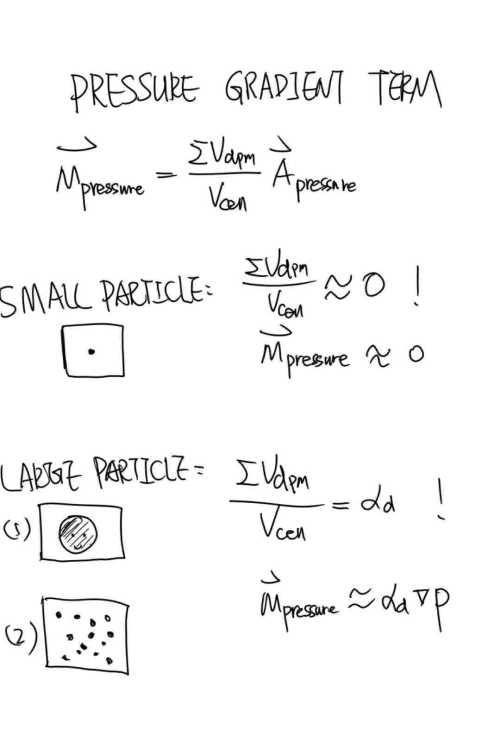好了，重新考虑方程\eqref{B}中右边的压力项有（$\rho_\rc=1$）：
\begin{equation}
-\nabla p_\rc - \bfM_\rd = -\nabla p_\rc + \alpha_\rd \nabla p_\rc = -\alpha_\rd \nabla p_\rc
\end{equation}
这样，方程\eqref{A}和方程eqref{B}就相符了。

# 结论

方程\eqref{A}和方程\eqref{B}的区别就在于考没考虑粒子的压力梯度项：

• 考虑粒子的压力梯度，则有相分数，对应方程\eqref{A}
• 没考虑粒子的压力梯度，则没有相分数，对应方程\eqref{B}

我在好多论文里面看到直接给出方程\eqref{A}和方程\eqref{B}，没有一点解释。是太过基础了么？总之，现在我明白了。

（虽然英文图，但我自己画的）

• 找到了个文章，有一点讨论：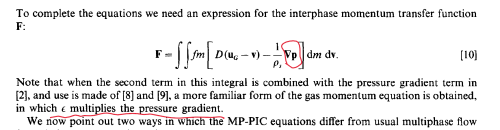The multiphase particle-in-cell (MP-PIC) method for dense particulate flows

1996年的MPPIC算法鼻祖文章，单篇被引300+，感兴趣的可以看看。

• 这篇参考文献有相关讨论：
Zhou, Z.Y., Kuang, S.B., Chu, K.W., Yu, A.B., 2010. Discrete particle simulation of particle–fluid flow: model formulations and their applicability. Journal of Fluid Mechanics 661, 482-510.

• @东岳 方程(3)和(4)是不是颠倒了？(1)推出(4)，(2)推出(3)。

• @linhan-ge 是的，已更正，目前正确了。

• PRESSURE GRADIENT TERM这个图中的$F=\frac{m}{V} \nabla p$，其中的$V$应该为$\rho$